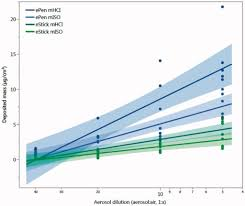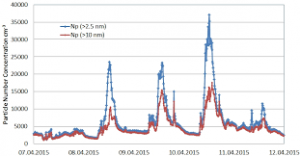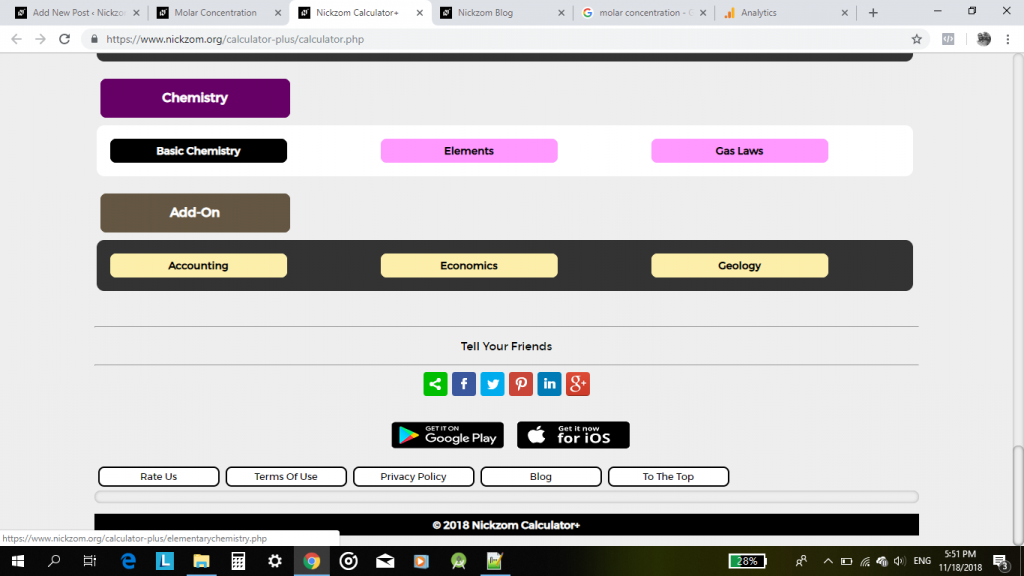## How to Calculate and Solve for the Molar Concentration, Molar Mass and Mass Concentration in Chemistry | The Calculator EncyclopediaThe image above represents the mass concentration.

To compute for mass concentration, two essential parameters are needed and these are molar concentration (c) and molar mass (M).

ρ = c x M

Where;
ρ = mass concentration
c = molar concentration
M = molar mass

Let’s solve an example;
Find the mass concentration when the molar concentration is 24 and molar mass is 15.

This implies that;
c = molar concentration = 24
M = molar mass = 15

ρ = c x M
ρ = 24 x 15
ρ = 360

Therefore, the mass concentration is 360 Kg/dm³.

Calculating the Molar Concentration when the Mass concentration and Molar Mass.

c = ρ / M

Where;
c = molar concentration
ρ = mass concentration
M = molar mass

Let’s solve an example;
Find the molar concentration when the mass concentration is 120 with a molar mass of 40.

This implies that;
ρ = mass concentration = 120
M = molar mass = 40

c = ρ / M
c = 120 / 40
c = 3

Therefore, the molar concentration is 3 mol/L.

## How to Calculate and Solve for the Number of Moles, Volume and Molar Concentration in Chemistry | Nickzom CalculatorThe image above represents the molar concentration.

To compute the molar concentration of a substance, two essential parameters are needed and these parameters are number of moles (n) and volume (V).

The formula for calculating the molar concentration:

c = nV

Where;
c = Molar Concentration
n = Number of moles
V = Volume

Let’s solve an example;
Find the molar concentration when the number of moles is 32 with a volume of 120.

This implies that;
n = Number of moles = 32
V = Volume = 120

c = nV
c = 32120
c = 0.266

Therefore, the molar concentration is 0.266 mol/L.

Calculating the Number of Moles when Molar Concentration and Volume is Given.

n = Vc

Where;
n = Number of moles
c = Molar Concentration
V = Volume

Let’s solve an example;
Find the number of moles when the molar concentration is 40 with a volume of 31.

This implies that;
c = molar concentration = 40
V = Volume = 31

n = Vc
n = 31 x 40
n = 1240

Therefore, the Number of Moles is 1240 mol.

## Nickzom Calculator Solves Molar Concentration in Chemistry

According to Wikipedia,

Molar concentration (also called molarityamount concentration or substance concentration) is a measure of the concentration of a chemical species, in particular of a solute in a solution, in terms of amount of substance per unit volume of solution. In chemistry, the most commonly used unit for molarity is the number of moles per litre, having the unit symbol mol/L. A solution with a concentration of 1 mol/L is said to be 1 molar, commonly designated as 1 M.

Nickzom Calculator+ (Professional Version) – The Calculator Encyclopedia requires two parameters to get the answer for a molar concentration in Chemistry such as:

• Number of Moles
• Volume

One can access Nickzom Calculator+ (Professional Version) – The Calculator Encyclopedia via any of these channels:

First, go to the calculator map, scroll down to the Chemistry section and click on Basic Chemistry.Now, click on Molar Concentration in Chemistry